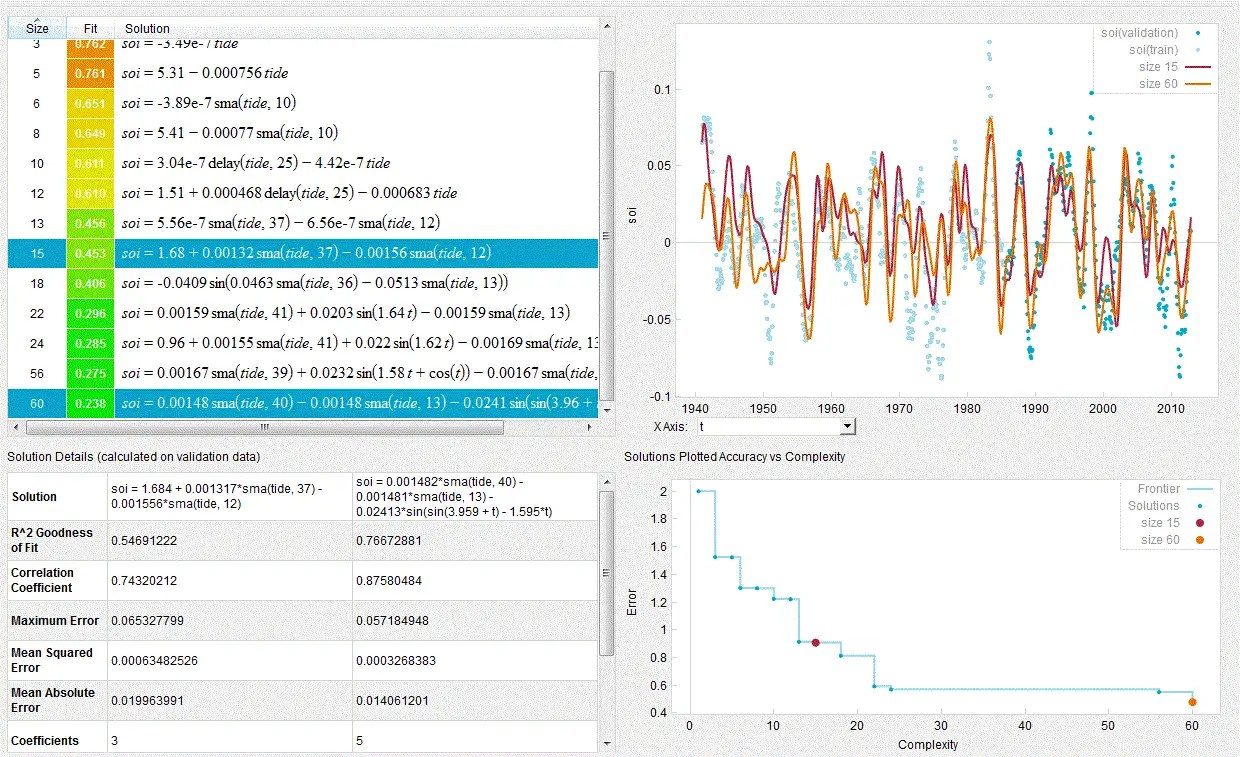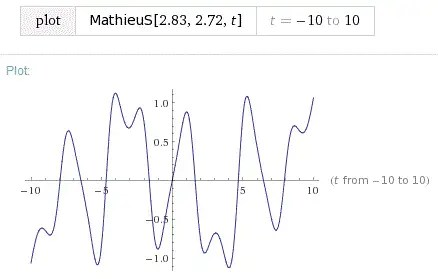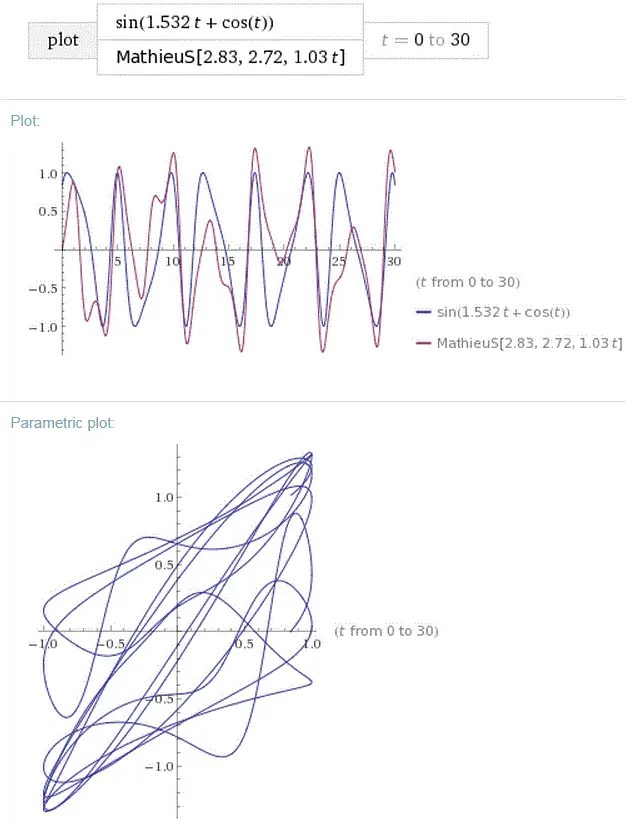# Two modes to ENSO Variability

[mathjax]Since the last post in the SOIM series, where we identified a highly correlated behavior in tide gauge data to the ENSO SOI measure, it occurred to me that many of the earlier entries in this series seemed to go down a different path.  That path still involved Mathieu differential equations, but the parameters differed and the right-hand side (RHS) forcing function did not play as significant a role (described initially here with a couple of follow up posts).

Yet from the first of the tide gauge posts there was a clear indication that an additional distinct sloshing mode might apply, and that this could be related to the “phantom” Mathieu waveform we may have been chasing earlier.

So the working formulation is the following composition, where f(t) is the phantom that we are trying to recover:

$$SOI(t) = g[ Tide(t) ] + f(t)$$

Using the Eureqa solver, we feed in the above equation to data covering a 100+ year time-span, and after a weekend of grinding away reveal the results below.  The main factor of course is the tide gauge data (modelled as a Mathieu sloshing with a strong biennial forcing), which contributes a 0.6 correlation coefficient once the long-term moving average is subtracted. But another factor further down the Pareto curve brings the correlation above 0.7.Fig 1 :  The SOI data was evaluated as a combination of the tide gauge data and some additional factor, f(t)

$$sin(1.532 r + cos(t))$$

which is essentially a modulated sine wave. Over a more recent time interval, the selection becomes more clear as the validation correlation coefficient approaches 0.88 for the combination of the factors.Fig 2: The results of Figure 1 operating in a shorter time interval

This is the scatter correlationFig 2a: Scatter correlation of the combined factors. The darker dots are training while the lighter dots are the validation values, which have a tighter spread approaching a correlation coefficient of 0.88.

Since we have recovered the phantom, we can now compare against what we had found earlier. In the initial post of this series, we identified a Mathieu parameter set from a crude linear regression fit as follows:

• a = 2.83
• q = 2.72
• T = 6.30 years (the Mathieu modulation term $omega = 2pi/T$ )

When we compare the discovered modulated sine wave, Figure 3, and a Mathieu function with the above parameter, Figure 4, we can immediately sense the similarity via the asymmetric and erratic waveform of the Mathieu functionFig 3 : Modulated sine waveFig 4 : Mathieu function

Overlaying the two we can see how a Mathieu function may be applicable for the modulated sine wave. The rationale is that since Eureqa does not generate Mathieu functions in its machine learning algorithm, it tries to do the best as it can, which is to create the unusually modulated sinusoid. The overlaid comparison between the two is shown in Figure 5. Note how the strong peaks line up, and the weaker inflections create the asymmetry.  The parametric plot correlation also clearly shows a 1:1 mapping in excursions.Fig 5 : (top) Overlay of modulated sine wave and Mathieu function (below) Correlation between the two waveforms

Importantly, the Mathieu function as shown is the response to an impulse, which is essentially a stimulus with a flat spectrum of input frequencies.  What we are seeing then is a quasi-periodic response that essentially is a stochastic resonance of the mode. The Mathieu waveform has a long repeat period that consists of multiple almost aperiodic cycles of the erratically jagged waveform.

This finding helps explain the earlier SOIM formulation that fit the SOI time series nicely but at the expense of a more complex set of dispersed quasi-biennial forcings.  That featured a Mathieu modulation frequency of about 0.8 rads/year.  The biennial model has a modulation frequency of 0.63 rads per year, while this second mode described in this post has a modulation frequency of 1 rads/year.   Note that what we were doing with the combined fit was trying to capture both modulations as one, and thus roughly averaging the two, or (0.63 + 1)/2 ~ 0.8 rads/year.

The role of the stratospheric quasi-biennial oscillation (QBO) may be up in the air, as this was used to established the quasi-biennial forcings and to understand a possible mechanism. The dispersed quasi-biennial forcing triplet was also used to fit paleo-climate proxy results here and here.  It is possible that the QBO may be more of a reflection of what ENSO is doing than an actual forcing function, as the true biennial function acts as a much better predictor of SOI. In other words, the biennial forcing used in the last post analysis is exactly a 2 year period, while the QBO is slightly dispersed around a value of 2.33 years. To find out if the 2.33 year period can be created out of a biennial forcing combined with a resonant frequency can be easily checked.  (note to self that a 1 rad/year is observed when comparing different strato layers).

# Discussion

There is a precedent for a multi-modal model of ENSO. The barely-cited(**) paper by Kim et al  describes 2 dominant modes with characteristics similar to the two modes outline above — what they characterize as a periodic biennial mode and an additional low-frequency stochastic mode.  Amazingly this pair provides a perfectly good working hypothesis (yea!) that we can draw from.  The previously described biennial forcing derived from the tide gauge data matches their biennial mode, and the additional 1 rad/year modulated Mathieu described in Figure 4 represents their low-frequency stochastic mode. It is stochastic only in the sense that an unforced response to a Mathieu function has a repeat period much longer than than the modulation period.

The Kim et al paper contains many interesting views of what they believe is occurring but do not lay out a numerical algorithm like we do with the SOIM differential equation, preferring to rely on empirical orthogonal functions (EOF) derived from empirical observations of the spatio-temporal measures of the ocean. They call this a cyclo-stationary EOF (CSEOF), which they claim captures the temporal aspects better by combining the deterministic and stochastic elements.Fig 6 : Kim et al CSEOF approach from 

They clearly dispute Peter Webster’s event model of ENSO, while siding more with Rasmussen’s biennial model.  They do suggest that the biennial mode is related to a delayed-action oscillator (see Azimuth Forum, where team members are evaluating this model) :

“The observational data seem to suggest that the biennial mode is strongly associated with the delayed-action oscillator hypothesis.”

The interpretation of the two modes in the Kim paper is worth referencing:

“In the context of CSEOF analysis, ENSO variability can be represented in terms of two dominant modes: the biennial mode and the low-frequency mode. The two modes have very different physical and dynamical mechanisms of evolution associated with them. As shown above, the biennial mode exhibits a strong physical evolution phase-locked to the annual cycle. Strong wave activity is evident in the extracted patterns of the sea level change and the thermocline fluctuation associated with the biennial mode. The low-frequency mode, on the other hand, does not show any significant physical evolution or a strong phase-locking tendency. The low-frequency mode appears to be a standing mode in the Pacific basin and its magnitude is determined primarily by the associated low-frequency stochastic undulation.
The separation of the two modes has an important implication for understanding the nature of ENSO and its predictability. For example, the biennial mode and the low-frequency mode have different natures of predictability. The deterministic component of evolution (tendency from a warm phase to a cold phase and vice versa) in the biennial mode is readily predictable, whereas the low-frequency mode is of a stochastic nature and its prediction is limited to its inherent predictability. Also, inter-ENSO variability can be explained in terms of an irregular interplay of the two modes. They are computationally independent modes, and henceforth, their evolution is independent of each other. As shown in Figure 5, the relative peak positions in the PC time series of the two modes are rather erratic, suggesting that the contribution of the two modes to SST change varies from one ENSO event to another. If the phases of the two modes are identical, then a stronger than normal ENSO event might be expected. If the two modes are out of phase, then an ENSO event will be weaker. The onset and termination times of El Niño and La Niña are also affected by the irregular interplay of the two modes; this may partially explain why ENSO events are loosely phase-locked to the annual cycle.”

Kim et al hold off on what is causing the second mode or even claiming that the biennial is strictly 2 years.

As one plausible cause, the 1 rad/year Mathieu modulation in the 2nd mode could be related to the Chandler wobble that we had identified earlier.  That too has a 1 rad/year frequency or 6.3 year beat period based on the precessional mode of 434 days. So while the biennial forcing is related to the rotational mode of the earth, the 2nd unforced mode is possibly related to the unforced precessional wobble of the axis.  Gross from JPL had previously identified the Chandler wobble with oscillations observed in the welling of the ocean .

That makes plausible sense to the degree that it certainly ties up some of the loose ends in what could have been thought as dead end excursions.  We are getting ever closer to a deterministic model of the quasiperiodic behavior of ENSO, and thus El Nino.

(**) The Kim paper only has 8 Google Scholar citations and most of these are self-cites.  Clearly this is not a well-known analysis.

## References

 Kim, Kwang-Yul, James J O’Brien, and Albert I Barcilon. “The Principal Physical Modes of Variability over the Tropical Pacific.” Earth Interactions 7, no. 3 (2003): 1–32.  PDF

 R. S. Gross, “The excitation of the Chandler wobble,” Geophysical Research Letters, vol. 27, no. 15, pp. 2329–2332, 2000.